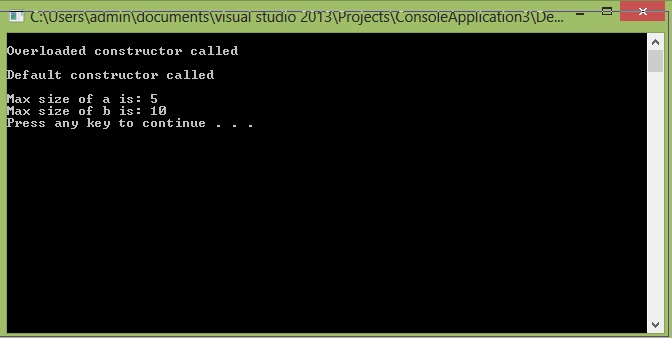# Constant members and Objects

In this part you will learn: 1. What are constant data members and functions 2. How to use them 3. Syntax in c++ 4. Program 5. Output Constant data members Constant data members are the data members of a class which once initialized, cannot be changed during any part of the program. Constant data members are declared just like normal data member declaration, the only difference is that we add a keyword ‘const’ before it. Constant data members help the programmers to perform checking of a program, they declare the members constant which they don’t want to change during program execution. Syntax
`class set{const int max_size;}`
Constant Objects Constant objects are the objects whose values cannot be changed after initialization. Like declaring a data member constant makes that particular data member constant, whereas declaring the whole object makes every member of the object constant. Sometimes programmers need a class whose value shouldn’t change during the whole execution of the program. One important thing about constant object is that constant objects can only be used in constant data functions. i.e. if we try to use constant objects in non-constant member functions, compiler will generate an error. Syntax
`int main(){const Class_Name Object_Name(parameters_if_any) ;//everything else}`
Program with constant data member In this example I have defined the member function of a Class. Basic Step:
`#include<iostream>#include<conio.h>using namespace std;`
These two are the most common and basic lines of a c++ program. The first one iostream is used to grant us most of the basic functions of C++ like Input and Output functions. The second one conio.h means console input output, the black screen we see is the console. Using namespace std; provides us an ease of using all the library functions without specifying that this function belongs to standard library. Without including it, we have to write ‘std’ with all the built-in functions. Class
`class set{public:	int *ele;	const int max_size = 10;	int size; 	set(){		cout << endl << "Default constructor called" << endl;		size = 0;		ele = new int[max_size];		} 	set(int m):max_size(m){		cout << endl << "Overloaded constructor called" << endl;		size = 0;		ele = new int[max_size];		} 	~set(){		cout << endl << "Destructor called" << endl;		if (size > 0)			delete[]ele;		ele = NULL;		}};`
In the above class “set “ I have made three member variables in which “ele” is a pointer to integer, size is the current size of array and max_size is a constant integer to store the maximum size of array made by pointer to integer “ele”. In the default and overloaded constructors, an array is being allocated of size (max_size). The point to observe is that the overloaded constructor is changing the value of max_size from default(10) to the value that will be passed in parameter. Later array will be allocated of that passed size. Let us take a look at the main program Main
`int main(){set a(5);set b; //a.max_size = 10;			//can't change the const valuecout << endl << "Max size of a is: " << a.max_size;cout << endl << "Max size of b is: " << b.max_size << endl;	system("pause");}`
In the main, first we are declaring an object “a” of class set and passing 5 as a parameter, i.e. we are giving the value of max_size at the time of initialization. After that we are making another object of same class named “b”, this object will have the max_size 10 which is set as default. In the commented part, we are trying to change the value of max_size of object “b” which is not possible as it is a constant data member. So the compiler won’t allow us to do so. Finally we are printing the values of data member “max_size” of class “a” and “b”, as a output we will see their values. Output